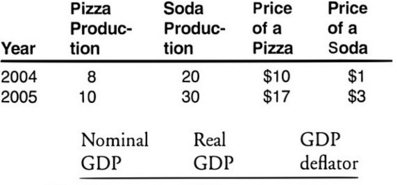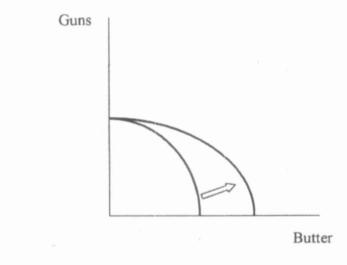# AP Macroeconomics Practice Test 9

### Test Information12 questions14 minutes

1. If the economy is in disequilibrium where the price level is such that the aggregate quantity of products demanded exceeds the aggregate quantity of products supplied, then

2. If 200 computers with a retail value of \$100,000 are domestically produced in 2005, but not sold until 2006, then GDP in 2005 is

3. Appropriate fiscal and monetary polices during the contractionary phase of the business cycle include

4. A change in government spending will have a greater short run impact on real output when

5. Suppose a country produces only two goods, pizza and soda. Given the information in the table below, nominal GDP, real GDP, and the GDP deflator in 2005 are (assume 2004 is the base year):6. If the economy experienced a decrease in real GDP and price level, this could best be explained by

7.The shift depicted in the diagram above could have been caused by

8. Suppose the real interest rate in a country rises. What can be expected to happen to the demand for this nation's currency and, therefore, the value of its currency and net exports?

Demand for currency      Value of currency      Net exports

9. According to Keynesian analysis, the Great Depression was caused by

10. According to Classical analysis, an economy in a recession can return to full employment through

11. If the cost of the basket of goods and services the Bureau of Labor Statistics uses to calculate the consumer price index rises from \$200 in the base period to \$400 in the current period, then the consumer price index

12. Contractionary monetary policy implies which of the following about the discount rate, the nominal interest rate, and aggregate demand?

Discount rate      Nominal interest rate      Aggregate demand